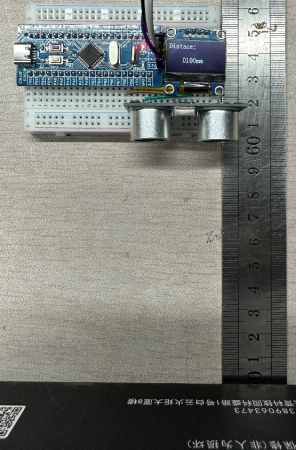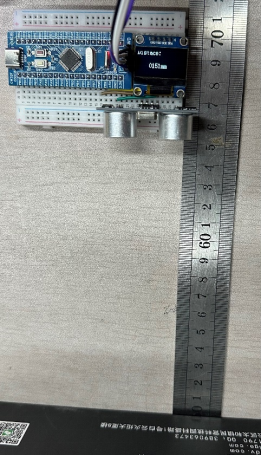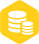# 超声波模块测距的使用方法

### 描述

一、简介

1. C-SR04超声波模块是一种常用的测距模块，其通过发射超声波并接收其反射信号来实现测距功能。因其成本低、精度高、使用简便等特点，被广泛应用于以下场景：

（1）避障机器人：HC-SR04超声波模块可以用于避障机器人的距离测量，通过检测障碍物与机器人的距离，实现避障控制。

（2）智能家居：HC-SR04超声波模块可用于智能家居中的人体检测和距离测量。例如，在门口安装超声波模块，可以检测人的接近并触发开门动作，或者用于室内的距离测量和触发自动照明等。

2. 本实验用到了CW32F030C8T6小蓝板、0.96寸OLED显示屏、HC-SR04超声波模块及Keil5开发环境。

使用流程   连接电源 将VCC引脚连接到+5V，GND引脚连接到GND。

三、核心代码

```HC_SR04.c:
#include "HC_SR04.h"

extern unsigned int time;

void HC_GPIO_Init(void)
{
__RCC_GPIOB_CLK_ENABLE();

GPIO_InitTypeDef GPIO_InitStruct;
GPIO_InitStruct.IT=GPIO_IT_NONE;
GPIO_InitStruct.Mode=GPIO_MODE_INPUT_PULLDOWN;//下拉输入
GPIO_InitStruct.Pins=GPIO_PIN_8;               //Echo
GPIO_Init(CW_GPIOB,&GPIO_InitStruct);

GPIO_InitStruct.Speed=GPIO_SPEED_HIGH;
GPIO_InitStruct.Mode=GPIO_MODE_OUTPUT_PP;     //推挽输出
GPIO_InitStruct.Pins=GPIO_PIN_9;               //Trig
GPIO_Init(CW_GPIOB,&GPIO_InitStruct);

PB09_SETLOW();   //Trig拉低，为输出脉冲触发信号做准备
}

unsigned int Measure_Distance(void) //测距
{
unsigned int distance=0;

SetTrig();     //10us的脉冲触发信号
Delay_us(10);
ResetTrig();
time=0;               //开始记录回波信号脉宽
distance=time*1.7;//根据声速和时间计算距离，即distance=time*340/2/100
/*
关于分辨力(mm)：
定时器每次对time加1是10us,10us=0.01ms,340m/s=340mm/ms
计算距离时，最小分辨力为：0.01(ms) * 340(mm/ms) / 2 = 1.7(mm)
小于模块标准精度3mm,故测距结果十分精准
*/
return distance; //返回距离,单位mm
}
main.c:
#include "HC_SR04.h"

extern unsigned int time;

void HC_GPIO_Init(void)
{
__RCC_GPIOB_CLK_ENABLE();

GPIO_InitTypeDef GPIO_InitStruct;
GPIO_InitStruct.IT=GPIO_IT_NONE;
GPIO_InitStruct.Mode=GPIO_MODE_INPUT_PULLDOWN;//下拉输入
GPIO_InitStruct.Pins=GPIO_PIN_8;               //Echo
GPIO_Init(CW_GPIOB,&GPIO_InitStruct);

GPIO_InitStruct.Speed=GPIO_SPEED_HIGH;
GPIO_InitStruct.Mode=GPIO_MODE_OUTPUT_PP;     //推挽输出
GPIO_InitStruct.Pins=GPIO_PIN_9;               //Trig
GPIO_Init(CW_GPIOB,&GPIO_InitStruct);

PB09_SETLOW();   //Trig拉低，为输出脉冲触发信号做准备
}

unsigned int Measure_Distance(void) //测距
{
unsigned int distance=0;

SetTrig();     //10us的脉冲触发信号
Delay_us(10);
ResetTrig();
time=0;               //开始记录回波信号脉宽
distance=time*1.7;//根据声速和时间计算距离，即distance=time*340/2/100
/*
关于分辨力(mm)：
定时器每次对time加1是10us,10us=0.01ms,340m/s=340mm/ms
计算距离时，最小分辨力为：0.01(ms) * 340(mm/ms) / 2 = 1.7(mm)
小于模块标准精度3mm,故测距结果十分精准
*/
return distance; //返回距离,单位mm
}```

四、实验最终现象审核编辑：汤梓红×
20